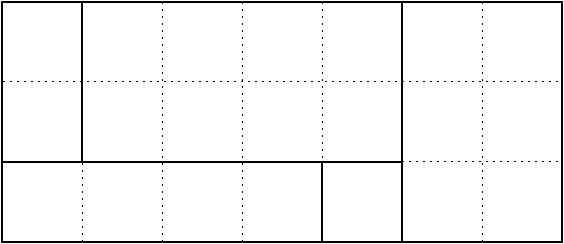$\def\bbN{{\mathbb N}} \def\bbQ{{\mathbb Q}} \def\bbR{{\mathbb R}} \def\bbZ{{\mathbb Z}}$
 © | Dror Bar-Natan: Classes: 2014-15: Math 475 - Problem Solving Seminar: (36) Next: Blackboards for Thursday March 5 Previous: Blackboards for Tuesday March 3

# Handout for March 5, "Argue by Contradiction", Pigeonhole

Reading. Sections 1.9 and 2.6 of Larson's textbook.

Next Quiz. Tuesday March 10 on these two sections and this handout.

Problem 1 (Larson's 2.5.13).

1. A derangement is a permutation $\sigma\in S_n$ such that for every $i$, $\sigma i\neq i$. Let $g_n$ be the number of derangements in $S_n$. Show that $g_1=0,\quad g_2=1,\quad g_n=(n-1)(g_{n-1}+g_{n-2}).$ Hint. A derangement interchanges $1$ with some other element, or not.
2. Let $f_n$ be the number of permutations in $S_n$ that have exactly one fixed point (namely, exactly one $i$ such that $\sigma i=i$). Show that $|f_n-g_n|=1$.Problem 2 (Larson's 2.6.3, modified). The points on a $3\times 7$ rectangular grid are coloured $Q$ and $M$. Show that you can find a rectangle among these points, whose sides are parallel to the grid axes, and all of whose corners are coloured the same way.

Problem 3 (off topic, but fun). A rectangle is said to be "part whole" if the length of at least one of its sides is a whole number. Prove that if a rectangle $R$ can be partitioned into part whole subrectangles, then $R$ is part whole.

Hint. Consider $\int e^{2\pi i(x+y)}dx\,dy$. (!?!!)

New! I just learned of an article that has 14 proofs of this result! Fourteen Proofs of a Result About Tiling a Rectangle by Stan Wagon, The American Mathematical Monthly 94-7 (1987) 601-617.

Problem 4 (Larson's 2.6.6). If 20 integers are chosen from within the elements of the arithmetic progression 1,4,7,...,100, show that you can find two of them whose sum is 104.

Problem 5 (Larson's 2.6.10, expanded).

1. Let $X$ be any real number. Prove that among the numbers $X,\ 2X,\ \ldots,\ (n-1)X$ there is one that differs from an integer by at most $1/n$.
2. Let $\alpha$ be an irrational number. Prove that the set $\{\{n\alpha\}\colon n\in\bbZ\}$ is dense in the unit interval $[0,1]$, where for a real number $x$, $\{x\}$ denotes its "fractional part" - the difference between $x$ and the largest integer $\lfloor x\rfloor$ smaller or equal to $x$.

Problem 6 (Larson's 2.6.11, modified).

1. Prove that in any group of six people there are either three mutual friends or three mutual strangers.
2. Prove that if all the edges and diagonals of a 17-gon are each coloured red, green, or blue, then you can find a single-colour triangle.
3. Can you generalize?

Problem 7 (Larson's 1.9.1). Given that $a$, $b$, and $c$ are odd integers, prove that the equation $ax^2+bx+c=0$ cannot have a rational root.

Problem 8 (Larson's 1.9.2). In a party with 2015 persons, among any set of four there is at least one person who knows each of the other three. It is also given that there are three people who do not know each other. Prove that the other 2012 people in the party know everyone at the party. (Assume that "knowing" is symmetric. What if not?)

Problem 9 (Larson's 1.9.5, abbreviated). A set $S$ of rational numbers is closed under addition and multiplication, and it is given that for every $r\in\bbQ$ exactly one of the following is true: $r\in S$, $-r\in S$, $r=0$. Prove that $S$ is the set of all positive rational numbers.

Problem 10 (Larson's 3.2.1 and 3.2.11, combined) Prove that any subset of size 55 of the set $\{1,2,3,\ldots,100\}$ must contains two numbers differing by 9, 10, 12, and 13, but not does need not contain a pair of numbers differing by 11.

Problem 11 (Larson's 3.2.17). Let $S$ be a set of primes such that $a,b\in S$ ($a$ and $b$ may be the same) implies that $ab+4\in S$. Prove that $S$ is empty.

Hint. It's nice to work mod 7.

Problem 12 (Larson's 5.4.1). Prove that $e=\sum_{k=0}^\infty\frac{1}{k!}$ is an irrational number.